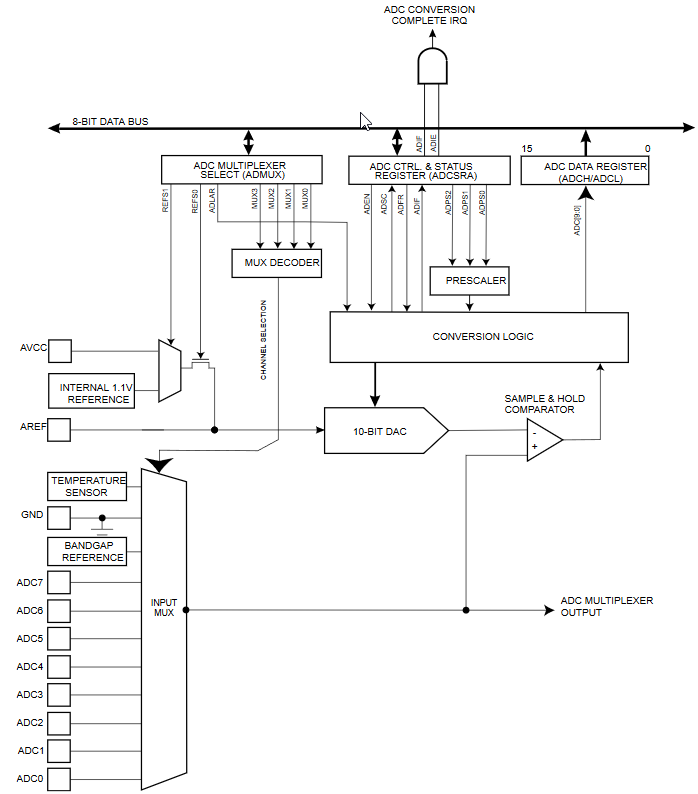# A/D converter - Measurement without blocking

Zápisník experimentátora

Hierarchy:

The function analogRead is programmed to block the microcontroller during measurement. During that time you can not do anything else. If you need to make better use of the microcontroller's time to process other tasks, it is advisable to modify the analog measurement so that it does not block the running of the program. In this example, we will write such a program.This is how analogRead looks like the current version of Arduino. I will only describe essential parts of the code.

• `if (pin >= 14) pin -= 14;` - On the board, Arduino has analog pins marked with A0-A6. The definitions are in the file pins_arduino.h. This calculation assures that the symbols move to index values of 0 and are then easily used in the function to select the specific pin on which the analogue measurement will be made.
• `sbi(ADCSRA, ADSC);` - Start A/D converter conversion. The start itself does not begin immediately. For measurement, the prescaler setting is used against the main frequency of the microcontroller and is normally set to 128. Analog measurement uses its own clock signal that is slowed down and conversion starts when the logic level changes. So, in worst conditions, the program will wait for 128 instructions until the conversion really starts. This is another reason to use non-blocking measurements.
• `while (bit_is_set(ADCSRA, ADSC));` - This is the critical line that blocks the entire program. Until the condition is met, the program will wait. Therefore, it is clear that we will concentrate in our source code especially on improving this line.
``````int analogRead(uint8_t pin)
{
uint8_t low, high;

#if defined(analogPinToChannel)
#if defined(__AVR_ATmega32U4__)
if (pin >= 18) pin -= 18; // allow for channel or pin numbers
#endif
pin = analogPinToChannel(pin);
#elif defined(__AVR_ATmega1280__) || defined(__AVR_ATmega2560__)
if (pin >= 54) pin -= 54; // allow for channel or pin numbers
#elif defined(__AVR_ATmega32U4__)
if (pin >= 18) pin -= 18; // allow for channel or pin numbers
#elif defined(__AVR_ATmega1284__) || defined(__AVR_ATmega1284P__) || defined(__AVR_ATmega644__) || defined(__AVR_ATmega644A__) || defined(__AVR_ATmega644P__) || defined(__AVR_ATmega644PA__)
if (pin >= 24) pin -= 24; // allow for channel or pin numbers
#else
if (pin >= 14) pin -= 14; // allow for channel or pin numbers
#endif

// the MUX5 bit of ADCSRB selects whether we're reading from channels
// 0 to 7 (MUX5 low) or 8 to 15 (MUX5 high).
ADCSRB = (ADCSRB & ~(1 << MUX5)) | (((pin >> 3) & 0x01) << MUX5);
#endif

// set the analog reference (high two bits of ADMUX) and select the
// to 0 (the default).
#if defined(__AVR_ATtiny25__) || defined(__AVR_ATtiny45__) || defined(__AVR_ATtiny85__)
ADMUX = (analog_reference << 4) | (pin & 0x07);
#else
ADMUX = (analog_reference << 6) | (pin & 0x07);
#endif
#endif

// without a delay, we seem to read from the wrong channel
//delay(1);

// start the conversion

// ADSC is cleared when the conversion finishes

// cause the results of each conversion to be discarded,
#else
// we dont have an ADC, return 0
low  = 0;
high = 0;
#endif

// combine the two bytes
return (high << 8) | low;
}``````

## Non-blocking voltage measurement

This is a modified code for measuring the voltage. The example is for pin A0 and Arduino Uno. In the function setup, we enable the analog measurement and set the clock signal prescaler to 128 (as Arduino does). Then we set the voltage reference to AVCC and pin A0.

We use the variable `adc_conversion_working` in the function loop. In it we have set whether we are doing analogue measurement. If the measurement is not active, we run the analogue measurement. We then periodically check the bit `ADSC` and at the moment it is not set, the analog measurement is finished. We will write the measured value to the serial port and wait for 500 ms.

Only when the condition is met, we perform an action at the end of measurement. The rest of the time the microcontroller can do other tasks.

``````const byte adcPin = A0; // = 14 (pins_arduino.h)

void setup() {
Serial.begin(115200);

| ((adcPin - 14) & 0x07); // Arduino Uno to ADC pin
}

void loop() {
}

// The ADC clears the bit when done
Serial.println(value);
delay(500);
}
}``````

## Source code

The source code is located on the GitHub server.

08.10.2018